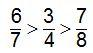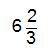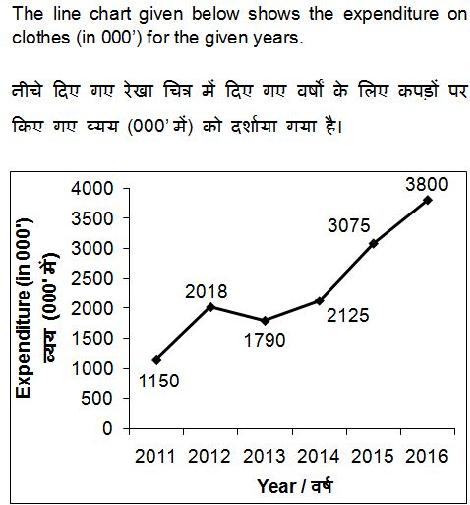# SSC MTS 2017 RE-EXAM PAPER : Held on 22-SEPTEMBER-2017 Shift-1(NUMERICAL APTITUDE)

SSC MTS 2017 RE-EXAM PAPER : Held on 22-SEPTEMBER-2017 Shift-1
(NUMERICAL APTITUDE)

QID : 26 - Which of the following is true?
1)2)3)4)QID : 27 -1) 8 + 3√7
2) 8 – 3√7
3) 16 + 3√7
4) 16 – 3√7
QID : 28 - If A = 23 x 34 and B = 25 x 32, then what is the value of A x B?
1) 28 x 36
2) 215 x 38
3) 28 x 38
4) 215 x 36
QID : 29 -1) Only I
2) Only II
3) Neither I nor II
4) Either I or II
QID : 30 -1) 4
2) 1.4
3) 1.3
4) 2.7
QID : 31 - How many two digit numbers are divisible by 9?
1) 9
2) 8
3) 10
4) 1
QID : 32 - P alone can complete a work in 10 days and Q alone can complete the same work in 20 days. If P and Q work together, then in how many days the same work will be completed?
1)2)3)4)QID : 33 - A and B together can complete a work in 8 days. B alone can complete the work in 24 days. In how many days A alone can complete the same work?
1) 16
2) 14
3) 12
4) 13
QID : 34 - What is the area (in cm2) of a square having perimeter 84 cm?
1) 361
2) 529
3) 484
4) 441
QID : 35 - Two successive discounts of 30% and 70% are given. What will be the net discount (in percentage)?
1) 63
2) 79
3) 100
4) 87
QID : 36 - After giving a discount of 40% on an article, there is still a profit of 25%. If no discount is given, then what will be the profit percentage?
1) 109.33
2) 25
3) 107.67
4) 108.33
QID : 37 - Marks of A and B are in the ratio 5 : 7 respectively. If the marks of A is 25, then find the marks of B?
1) 60
2) 35
3) 28
4) 45
QID : 38 - If A : B = 4 : 5 and B : C = 20 : 11, then find A : B : C ?
1) 4 : 20 : 7
2) 16 : 25 : 22
3) 16 : 20 : 11
4) 8 : 10 : 7
Correct Answer: 16 : 20 : 11
QID : 39 - Average of 43, 57, 68, 32, 97 and x is 63. What is the value of x?
1) 83
2) 77
3) 81
4) 75
QID : 40 - If the ratio of selling price and cost price is 3 : 4 respectively, then what is the loss percentage?
1) 10
2) 33.33
3) 20
4) 25
QID : 41 - An article is sold at 30% loss. If the selling price is increased by 50%, then what is the profit percentage?
1) 5
2) 8
3) 12
4) 20
QID : 42 - What is the value of 20% of 30% of 7200?
1) 480
2) 432
3) 412
4) 360
QID : 43 - 12% of what number is equal to 30% of 960?
1) 2400
2) 1720
3) 1440
4) 2880
QID : 44 - If a car travels a distance with 20% less speed, then it will reach 15 minutes late. What is the usual time (in minutes) taken by the car to travel the same distance?
1) 80
2) 90
3) 75
4) 60
QID : 45 - A goes from Point X to Point Y at a speed of 60 km/hr and comes back with a speed of 80 km/hr. What is the average speed (in km/hr) of A for going and coming back?
1) 66.66
2) 68.57
3) 67.33
4) 69.43
QID : 46 - A certain amount double in 5 years, when invested at simple interest. In how many years will it become 8 times?
1) 35
2) 40
3) 30
4) 45
QID : 47 -Expenditure in year 2016 is how much percent more than the expenditure in year 2015?
1) 19.07
2) 23.57
3) 21.16
4) 17.64
QID : 48 -What is the average expenditure (in 000’) per year from the year 2014 to 2016?
1) 3120
2) 3080
3) 3000
4) 3430
QID : 49 -Expenditure in the year 2014 is what percent of the expenditure in year 2013?
1) 118.71
2) 18.71
3) 115.76
4) 15.76